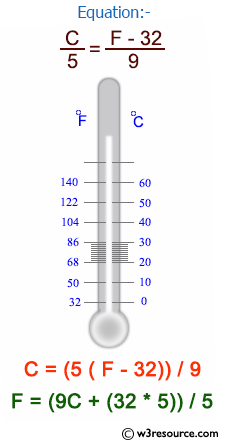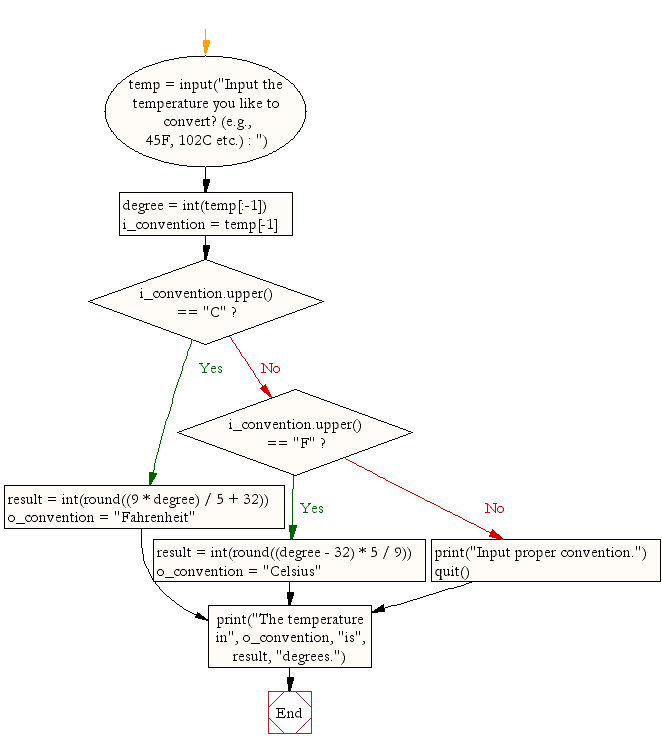﻿ Python Exercise: Convert temperatures to and from celsius, fahrenheit - w3resource# Python Exercise: Convert temperatures to and from celsius, fahrenheit

## Python Conditional: Exercise-2 with Solution

Write a Python program to convert temperatures to and from celsius, fahrenheit.

Python: Centigrade and Fahrenheit Temperatures :

The centigrade scale, which is also called the Celsius scale, was developed by Swedish astronomer Andres Celsius. In the centigrade scale, water freezes at 0 degrees and boils at 100 degrees. The centigrade to Fahrenheit conversion formula is:

Fahrenheit and centigrade are two temperature scales in use today. The Fahrenheit scale was developed by the German physicist Daniel Gabriel Fahrenheit . In the Fahrenheit scale, water freezes at 32 degrees and boils at 212 degrees.

`C = (5/9) * (F - 32)`

where F is the Fahrenheit temperature. You can also use this Web page to convert Fahrenheit temperatures to centigrade. Just enter a Fahrenheit temperature in the text box below, then click on the Convert button.Sample Solution:-

Python Code:

``````temp = input("Input the  temperature you like to convert? (e.g., 45F, 102C etc.) : ")
degree = int(temp[:-1])
i_convention = temp[-1]

if i_convention.upper() == "C":
result = int(round((9 * degree) / 5 + 32))
o_convention = "Fahrenheit"
elif i_convention.upper() == "F":
result = int(round((degree - 32) * 5 / 9))
o_convention = "Celsius"
else:
print("Input proper convention.")
quit()
print("The temperature in", o_convention, "is", result, "degrees.")
```
```

Sample Output:

```Input the  temperature you like to convert? (e.g., 45F, 102C etc.) : 104f
The temperature in Celsius is 40 degrees.
```

Flowchart:Python Code Editor:

Have another way to solve this solution? Contribute your code (and comments) through Disqus.

What is the difficulty level of this exercise?

Test your Python skills with w3resource's quiz

﻿

## Python: Tips of the Day

Returns a list with n elements removed from the beginning

Example:

```def tips_take(itr, n = 1):
return itr[:n]
print(tips_take([1, 2, 3], 5))
print(tips_take([1, 2, 3], 0))
```

Output:

```[1, 2, 3]
[]
```